## Modify Path

The effects in this section all modify an existing path.

Adds nodes to a path, leaving the shape of the path unchanged. The nodes are added evenly with a minimum specified spacing. Good for creating scissor lines.

Convert regular shapes to paths before applying.Example of using Add Node. Top: A Bezier curve defined by three nodes. Middle: Same curve after using Add Node. Bottom: Curve converted to cut line.

### Color Markers to Match Stroke

Automates changing the Markers to match the color of the Stroke. The effect makes copies of a Markers in the <defs> section of the SVG file with the appropriate color. This effect is a temporary work-around until the SVG 1.2 standard is finalized. The new standard is expected to simplify matching Marker color with Stroke color.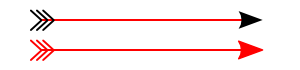Example of using the Color Marker to Match Stroke effect. The top arrow shows the markers just after changing the stroke color. The bottom arrow shows the results of applying this effect.

### Edge 3D

New in v0.46.

Adds highlights and shadows to simulate 3D objects like buttons. The effect works by adding paths that are blurred via the Gaussian Blur filter and then clipped. The paths have partial transparency, with white for highlights and black for shadows. The effect only works on paths so regular shapes need to be converted first. You may also need to add additional nodes.

Parameters:

• Illumination Angle: Sets the angle from which light arrives.
• Shades: Sets the number of different gray levels. The path is broken up into sections according to the relative angles of the nodes. A shading path is generated for each level. You must have a least as many nodes in the original path as shades.
• Only black and white: If checked, only the lightest (white) and darkest (black) parts are generated.
• Stroke width: Not used (fixed internally at 10).
• Blur stdDeviation: Sets the blur radius for the Gaussian Blur filter in pixel units.
• Blur width, Blur height: Sets the width and height of the Filter Effects Region, the area where the blur is calculated. These parameters are not very useful (you can not set the x and y terms). Leave both set at 2.0.Example of using Edge 3D. Both square and circle were converted to paths. The Add Node effect was used to add nodes to the circle. The number of Shades is 2 for the square and 4 for the circle.

### Envelope

Modified slightly in v0.46.

Broken in v0.44 and v0.45. Can be fixed by removing the line "self.q['y'] = docheight - self.q['y'] - self.q['height']" in `share/inkscape/extensions/summersnight.py`.

Distorts a path so that the path's original bounding box is mapped to the edges of a quadrilateral. To use the effect, select the path to transform first, then add the quadrilateral path to the selection (this order is the inverse of what was used before v0.46). Regular shape objects must be converted to a path before transformation.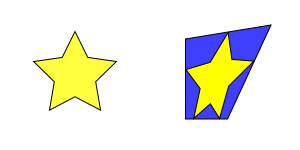Left: Original path. Right: Path after mapping bounding box to quadrilateral.

The effect works on a single path. The beginning point of the quadrilateral path will determine the orientation of the transformed image. If the image is inverted (like looking through a mirror), reverse the direction of the quadrilateral path with the PathReverse (Shift+R) command.

This effect could be used to produce a pseudo-perspective effect. For real perspective, use the Perspective effect.

### Flatten Bezier

This effect converts selected Bezier curves to an approximation composed of straight-line paths. The number of line segments used is determined by the Flatness parameter. The smaller the Flatness, the more line segments are used.Example of using Flatten Bezier. Top: A Bezier curve defined by three nodes. Middle: After using effect with Flatness of 10. Bottom: After using effect with Flatness of 2.

### Fractalize

This effect turns a straight-line segment into a crooked segment. It works by finding the midpoint of the line segment, adding a node at that point, and then moving the node a random distance perpendicular to the original path direction. This division routine is called recursively depending on the setting of the Subdivisions entry in the dialog, doubling the number of resulting segments for each increase by one. The Smoothness of the path can also be specified. The magnitude of the perpendicular displacement is a random function with the limits determined by ±(Segment length)/(1+Smoothness).

The effect will also work on a curved path by turning the path into a series of line segments between the path's nodes.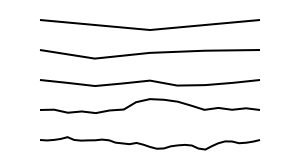Example of using Fractalize. From top to bottom, the Subdivision parameter was increased from 1 to 5. The Smoothness parameter was set to 10.

### Jitter Nodes

Previously named Radius Randomizer.

Randomly shift nodes and/or node handles. The Maximum displacement parameter controls the magnitude of the randomization. When the Use normal distribution box is not checked, the magnitude of displacements will be uniformly distributed between zero and the Maximum displacement value (in pixels). When the box is checked, the magnitudes of the displacements will have a Normal (a.k.a. Gaussian or Bell curve) distribution with a standard deviation of 0.5 times the Maximum displacement value. The Normal distribution option may produce more natural-looking variations in a path. In both cases, the direction of the displacement will be random.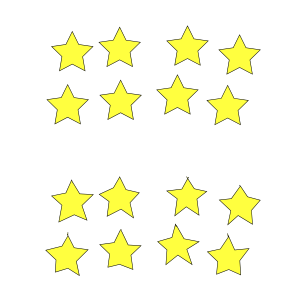Top: Array of stars created with the Create Tiled Clones dialog. Bottom: Same array after applying the Jitter nodes effect. Note that the stars must be converted to paths before the effect is applied.

### Fractal (Koch)

Removed in v0.45. Use the L-System effect for creating fractals like Koch's Snowflake. Use the Pattern along Path effect for putting objects along a path.

This effect allows the drawing of figures inspired by Helge von Koch's snowflake. Using the script requires two steps. The first step is to select a path and run the Fractal (Koch) - Load Pattern script. This is the path segment that will be used to replace the paths in the target object.

The second step is to select the target object and to run the Fractal (Koch) script. The object should consist of a path with a number of line segments. Each line segment between adjacent nodes will be replaced by the path loaded in the first step, scaled to fit. Regular shaped objects must be converted to a path first.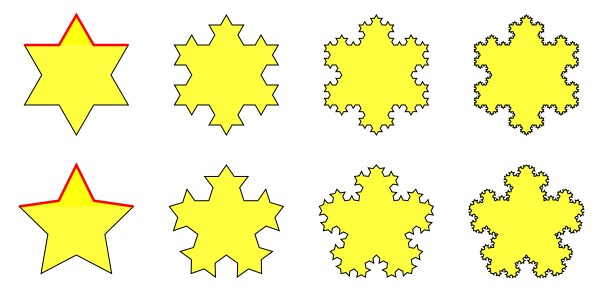Top: The classic Koch's snowflake. The red line segment is that loaded in the first step. (Properly, the series should start with an equilateral triangle.) Bottom: A variation of Koch's snowflake starting with a five-sided star. The leftmost column has the original target objects. In the columns to the right, the effect is applied one, two, and three times.

The Koch effect also allows one to produce interesting borders.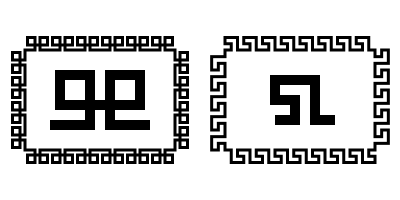Borders produced by using the Koch effect on a rectangle that has had additional nodes added using the Add Node effect. The pattern loaded is shown in the center of each border.

### Perspective

New in v0.45.

Distorts a path (or a Group of paths) so that the path's original bounding box is mapped to the edges of a quadrilateral and a perspective effect is applied to the path. To use the effect, select a quadrilateral first, then select the path to transform. Regular shapes and text must be converted to a path before transformation. This effect requires the Numpy (Numerical Python) package.

The beginning point of the quadrilateral path will determine the orientation of the transformed image; in most cases, the quadrilateral path should be started from the lower-left corner and proceed in a clockwise direction.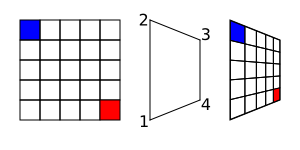Left: Original path. Middle: Target quadrilateral. The nodes have been numbered to show the normal order in which they should be drawn. Right: Path after mapping bounding box to quadrilateral.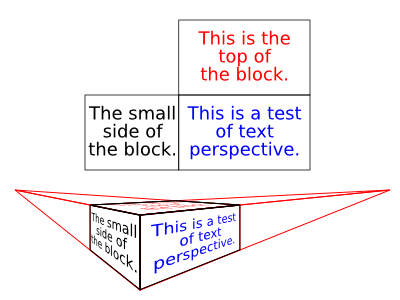Mapping text to the sides of a block with perspective. The text must be converted to a path. In order to center the text, the text has been grouped with a rectangle (also converted to a path).

Renamed Jitter Nodes in v0.45.

### Rubber Stretch

New in v0.46.

Distorts a path as if the path was stretched vertically or the path was squeezed horizontally. The amount of the distortion is controlled by the Strength and Curve parameters. Adding extra nodes may produce a better result.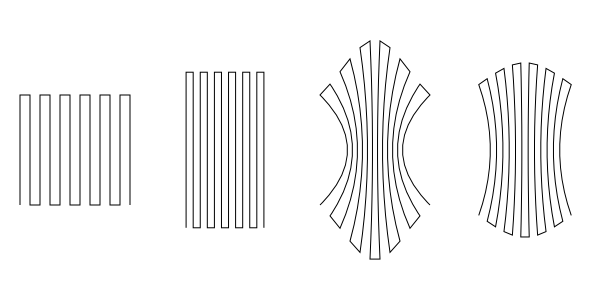From left to right: Input path (with extra nodes added). After applying the effect with parameters values for Strength and Curve of 50%, 0%; 0%, 50%; and 25%, 25% respectively.

### Straighten Segments

Reduces the curvatures of path segments. The amount of straightening can be specified. Behavior 1 moves the node handles toward the nodes, Behavior 2 moves the node handles to a point 1/3 of the distance between the neighboring node away from the node. There is little visual difference between these two options.From top to bottom, a path with the Straighten Segment effect applied 0, 1, 2, and 3 times.

### Whirl

This effect twists an object around a point, like what might happen if you dropped things in whirling water (except the farther away form the center, the greater the displacement for this effect). The amount of “whirling” and the center of the whirl are input parameters (v0.45). Objects must be converted to paths before applying the effect.

Note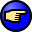v0.45: The center is defined in SVG coordinates where the y axis is inverted. To find the correct center, subtract the desired y coordinate from the page height. v0.46: The center of view is used for the center of the whirl. To whirl around the center of an object, select the object and then use View → Zoom →Selection (3) to center the view on the object.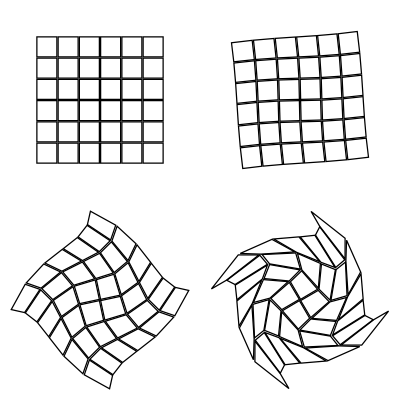From left to right, top to bottom: Whirl of 0, 1, 10, and 25 units. In each case, the center of the whirl is the center of each array of small squares.